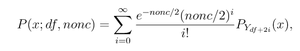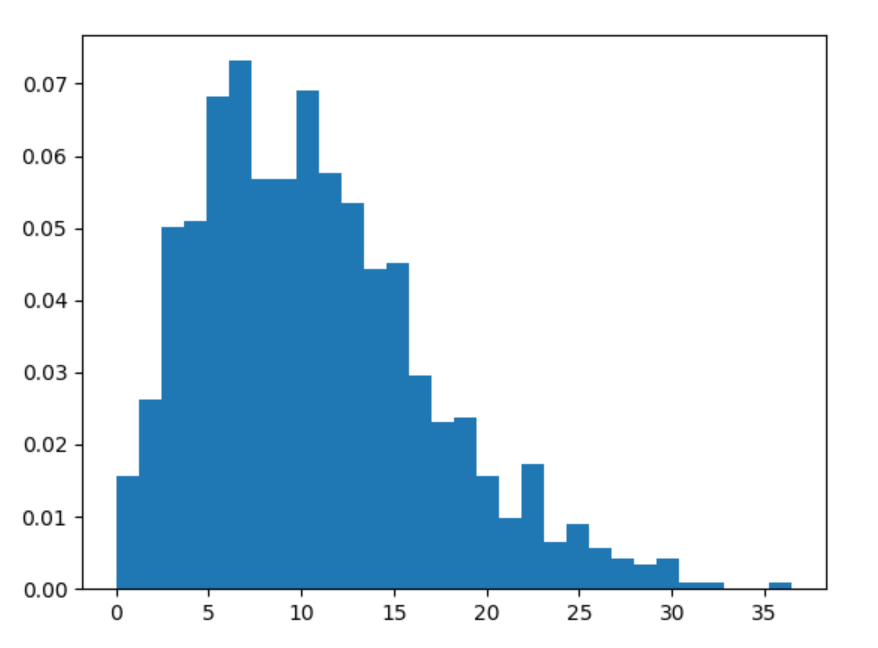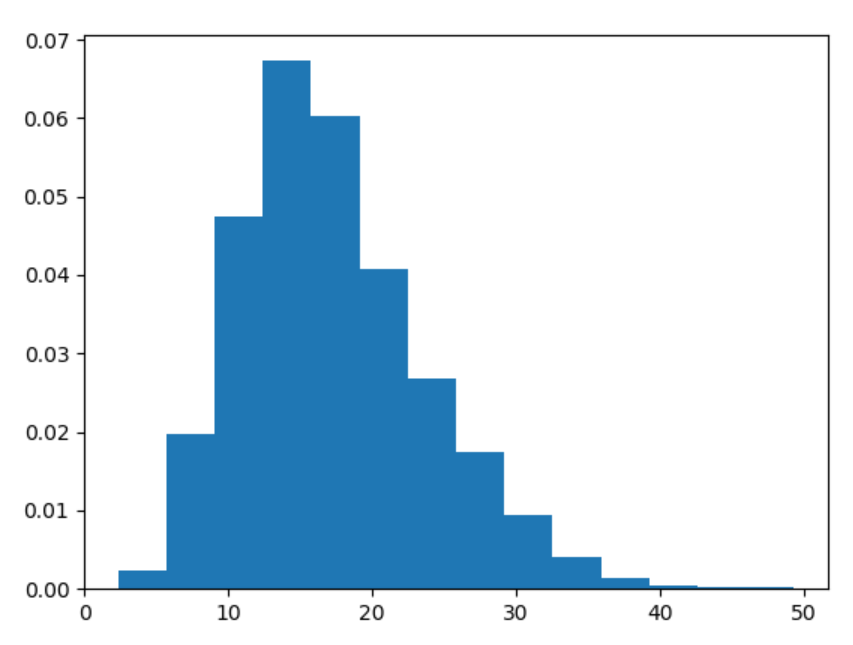# numpy.random.noncentral_chisquare() in Python

• Last Updated : 15 Jul, 2020

With the help of numpy.random.noncentral_chisquare() method, we can get the random samples of noncentral chi-square distribution and return the random samples of it by using this method.noncentral chi-square distribution

Syntax : numpy.random.noncentral_chisquare(df, nonc, size=None)

Return : Return the random samples as numpy array.

Example #1 :

In this example we can see that by using numpy.random.noncentral_chisquare() method, we are able to get the random samples of noncentral chi-square distribution and return the random samples by using this method.

## Python3

 `# import numpy``import` `numpy as np``import` `matplotlib.pyplot as plt`` ` `# Using noncentral_chisquare() method``gfg ``=` `np.random.noncentral_chisquare(``1.21``, ``9.89``, ``1000``)`` ` `count, bins, ignored ``=` `plt.hist(gfg, ``30``, density ``=` `True``)``plt.show()`

Output :Example #2 :

## Python3

 `# import numpy``import` `numpy as np``import` `matplotlib.pyplot as plt`` ` `# Using noncentral_chisquare() method``gfg ``=` `np.random.noncentral_chisquare(``14.05``, ``3.24``, ``3000``)`` ` `count, bins, ignored ``=` `plt.hist(gfg, ``14``, density ``=` `True``)``plt.show()`

Output :My Personal Notes arrow_drop_up